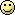# Aargh! How do you add two floats?

This has now been resolved. Use bcmath functionsThe following code prints “FAILED!” and I can’t figure out why.

``````

\$foo1     =  2.8;
\$foo2     =  3.15;
\$expected =  5.95; // foo1 + foo2

\$actual   =  \$foo1 + \$foo2;

if(\$actual == \$expected){
print 'It worked!!!';
}else{
print 'FAILED!';
}

``````

What is strange is that the running the script with the following values prints “It worked!!!”

``````

\$foo1     =  2.8.;
\$foo2     =  3.13;
\$expected =  5.93;

``````

This also works -

``````

\$foo1     =  2.85;
\$foo2     =  3.1;
\$expected =  5.95;

``````

This could be an issue with my install of PHP. I’m running the default dotdeb (PHP 5.2.0 with suhoshin patch) install on Debian Sarge.
OR maybe I’m just too tiredI also searched the PHP Bug database but couldn’t find anything

Any help will be appreciated.

/Saurabh

You are adding two floats, thus values aren’t precise but approximate. You should define an own precision of calculations:

``````
if ((\$a >= \$b - \$eps) && (\$a <= \$b + \$eps)) {
...
}

``````

Or calculate and use the machine epsilon.

So never trust floating number results to the last digit and never compare floating point numbers for equality. If you really need higher precision, you should use the arbitrary precision math functions or gmp functions instead.

Hope that helpsBTW, this is my 100th post!

Thanks pkSML this is solved!im gonna have to test this, but surely that calculation would work if you place quotes around the \$foo1 \$foo2 and \$expected values??
i apologise in advance if im wrong

Not always, 8.10 is sometimes stored as 8.09999999…

im gonna have to test this, but surely that calculation would work if you place quotes around the \$foo1 \$foo2 and \$expected values??
i apologise in advance if im wrong

No, not necessarily. Floating point numbers are dealt with differently depending upon CPU. This becomes extremely more apparent the more places you have to the right of the point. Making the number a string doesn’t change the fact that PHP calculated it to be a different value to what you expected.

This is something that must be taken into consideration when writing code to be used across platforms since all platforms will give different results. Interestingly, Java doesn’t suffer this cross-platform problem since the VM actually does some of the math, not just the CPU - with the HUGE drawback of being hundreds of times slower at doing math with floating point numbers.

I decided to perform this test myself. I have a server with PHP v5.1.4 running on Windows XP.

Here’s my code:

``````<PRE>
<?php
\$foo1     =  2.8;
\$foo2     =  3.15;
\$expected =  5.95; // foo1 + foo2

\$actual   =  \$foo1 + \$foo2;

if(\$actual == \$expected){
print 'It worked!!!';
}else{
print 'FAILED!';
}
echo "\
foo1 = \$foo1\
";
echo "foo2 = \$foo2\
";
echo "expected = \$expected\
";
echo "actual = \$actual\
";
?>
</PRE>
``````

Output:

``````FAILED!
foo1 = 2.8
foo2 = 3.15
expected = 5.95
actual = 5.95
``````

So you can see that the if statement didn’t work right, but the mathematical computation worked flawlessly for me.